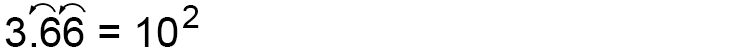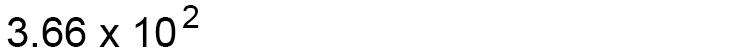# Standard (index) form – Turn into indices

Standard (index) form is expressing large or small numbers into indices.

It is always written in the form:

Axx10^nStandard forms (Standard form) can be thrown in the seas (indices) said the new law.

The rules when writing a number in standard form is that first you write down the number between 1 and 10, then you write x10 (to the power of a number.)

A mnemonic to remember the rule of standard form is as follows:

It was standard for the class form (standard form) to write down one number and hold it up.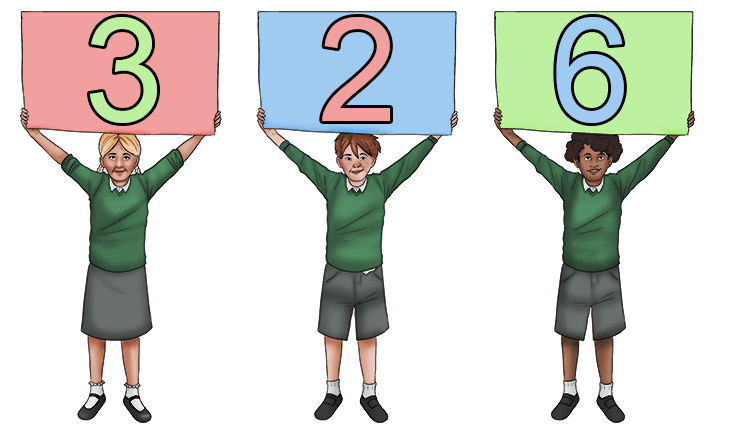Example

Change 366 to standard form.

The rule is to write down the number between 1 and 10.
Here it is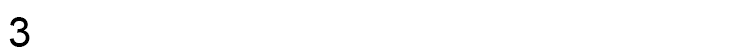Then you write x10 to the power.
This becomes: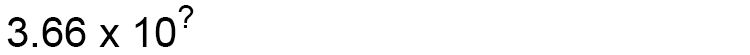Which would be: